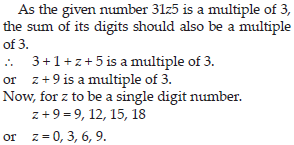# Playing With Numbers (Mathematics) Class 8 - NCERT Questions

Q 1.

Find the values of the letters in each of the following and give reasons for the steps involved.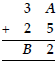SOLUTION: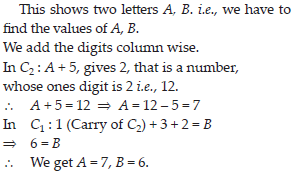Q 2.

Find the values of the letters in each of the following and give reasons for the steps involved.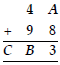SOLUTION: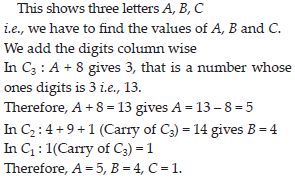Q 3.

Find the values of the letters in each of the following and give reasons for the steps involved.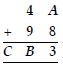SOLUTION: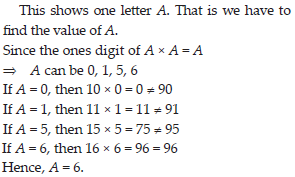Q 4.

Find the values of the letters in each of the following and give reasons for the steps involved.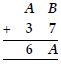SOLUTION: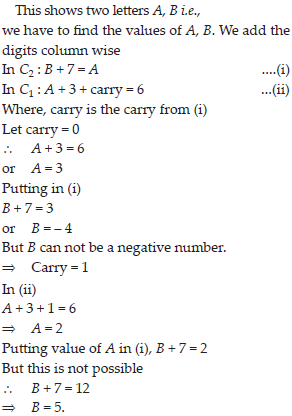Q 5.

Find the values of the letters in each of the following and give reasons for the steps involved.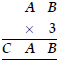SOLUTION: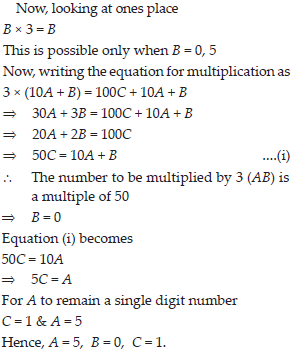Q 6.

Find the values of the letters in each of the following and give reasons for the steps involved.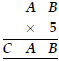SOLUTION: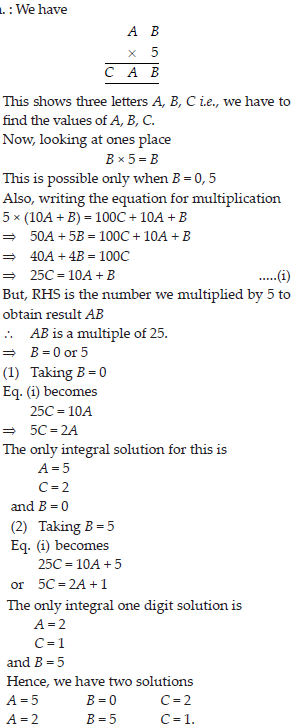Q 7.

Find the values of the letters in each of the following and give reasons for the steps involved.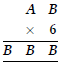SOLUTION: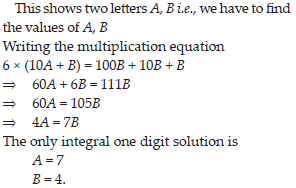Q 8.

Find the values of the letters in each of the following and give reasons for the steps involved.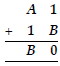SOLUTION: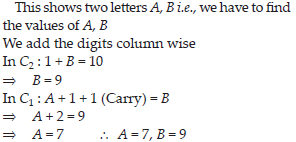Q 9.

Find the values of the letters in each of the following and give reasons for the steps involved.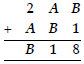SOLUTION: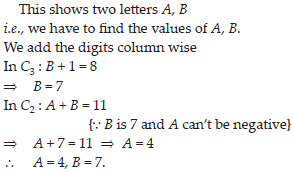Q 10.

Find the values of the letters in each of the following and give reasons for the steps involved.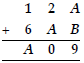SOLUTION: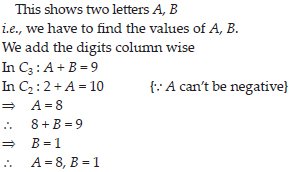Q 11.

If 21y5 is a multiple of 9, where y is a digit, what is the value of y?

SOLUTION: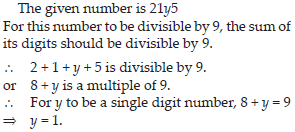Q 12.

If 31z5 is a multiple of 9, where z is a digit, what is the value of z? You will find that there are two answers for the last problem. Why is this so?

SOLUTION: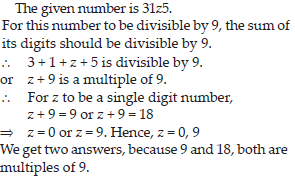Q 13.

If 24x is a multiple of 3, where x is a digit, what is the value of x?
(Since 24x is a multiple of 3, its sum of digits 6 + x is a multiple of 3; so 6 + x is one of these numbers : 0, 3, 6, 9, 12, 15, 18, ........ . But since x is a digit, it can only be that 6 + x = 6 or 9 or 12 or 15. Therefore, x = 0 or 3 or 6 or 9. Thus, x can have any of four different values).

SOLUTION: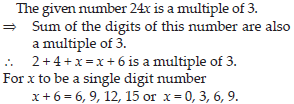Q 14.

If 31z5 is a multiple of 3, where z is a digit, what might be the values of z?

SOLUTION: